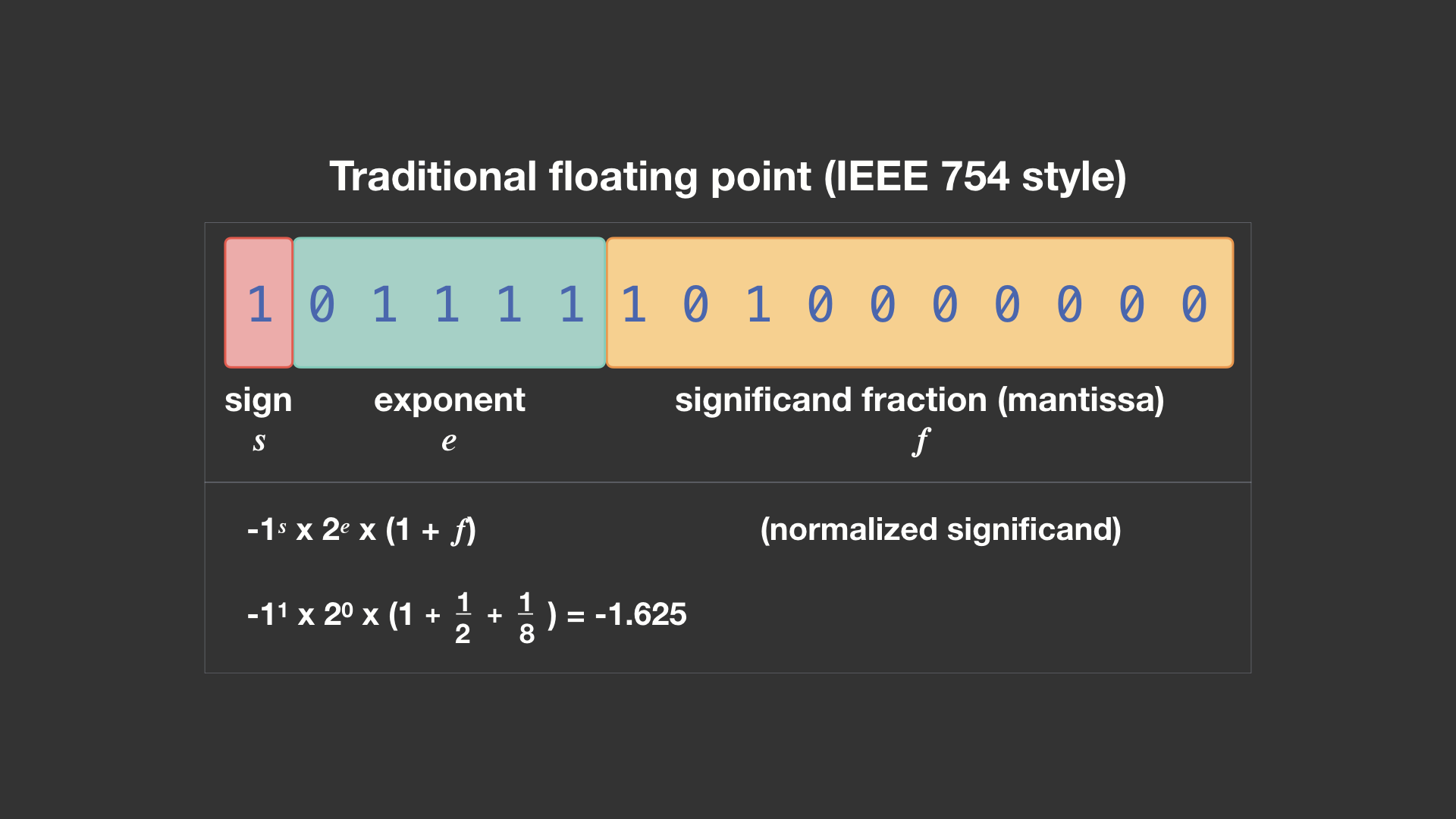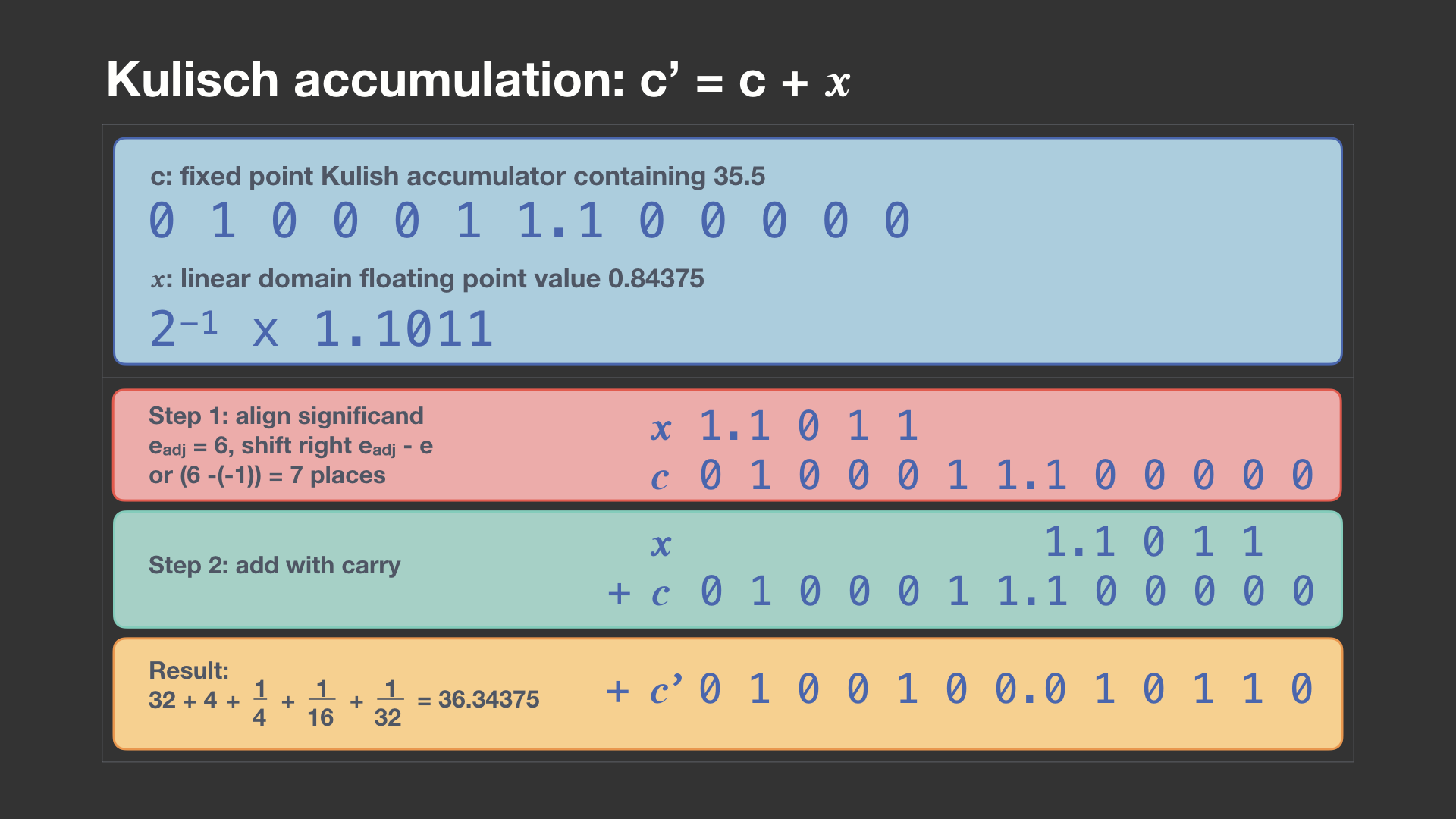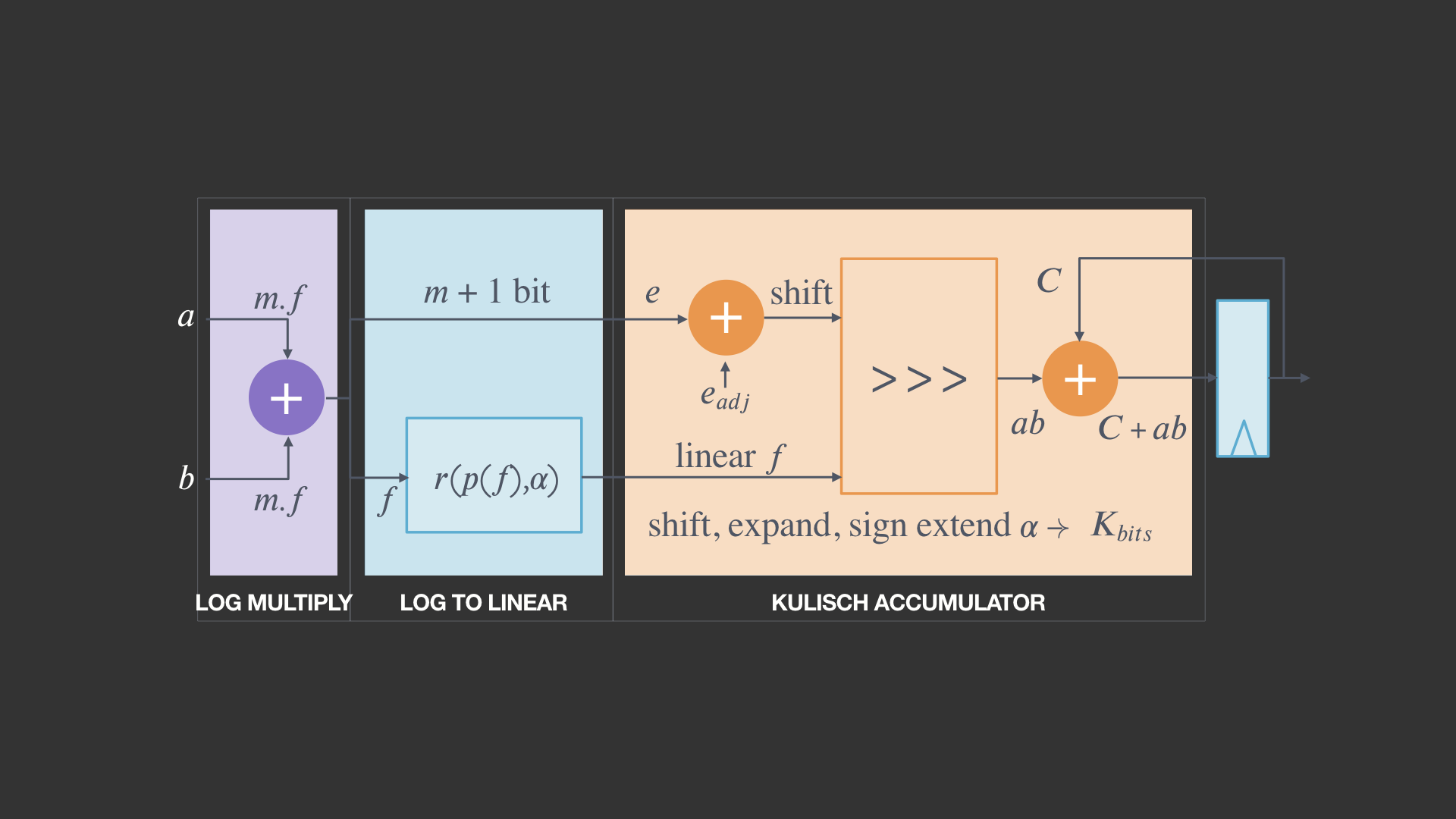In recent years, compute-intensive artificial intelligence tasks have prompted creation of a wide variety of custom hardware to run these powerful new systems efficiently. Deep learning models, such as the ResNet-50 convolutional neural network, are trained using floating point arithmetic. But because floating point has been extremely resource-intensive, AI deployment systems typically rely upon one of a handful of now-standard integer quantization techniques using int8/32 math.

We have developed an alternate approach to making AI models run efficiently. Building on a lineage of ideas reaching back to the early days of computer science more than 70 years ago, our method optimizes floating point itself.

We have made radical changes to floating point to make it as much as 16 percent more efficient than int8/32 math. Our approach is still highly accurate for convolutional neural networks, and it offers several additional benefits:

• Our technique can improve the speed of AI research and development. When applied to higher-precision floating point used in AI model training, it is as much as 69 percent more efficient.
• Today, models are typically trained using floating point, but then they must be converted to a more efficient quantized format that can be deployed to production. With our approach, nothing needs to be retrained or relearned to deploy a model. AI developers can thus deploy efficient new models more easily.
• Integer quantization schemes today are growing ever more complicated and in some cases might be “overfitting” on a particular task (and thereby not retaining their general-purpose application). An efficient, general-purpose floating point arithmetic that preserves accuracy can avoid this issue.

Our techniques are discussed in detail in the research paper “Rethinking floating point for deep learning.” It will take time to develop new chips designed to perform floating point math with these techniques. But the potential benefits include faster AI computation in data centers, lower-power designs for better AI on mobile devices, and simple, faster ways to achieve performance goals with fewer software changes. The slowdown of Moore’s Law and the age of “dark silicon” is at hand. Continued performance gains will require rethinking low-level hardware design decisions made decades ago, such as the IEEE 754 floating point standard, and use of mathematical approximation where applicable. Neural networks in particular provide an excellent opportunity for this reevaluation, as they are quite tolerant of variation and experimentation.

Our hardware designs for ASIC/FPGA and C++/PyTorch code for its evaluation are now publicly available to the AI community. We hope that the AI community will join us in exploring this new approach.

Engineers who work in other fields may not be familiar with how traditional floating point would compare with our alternatives, so a brief summary may be helpful. As is commonly known, floating point can represent both large and small real numbers in a reasonable amount of computer storage, using a system that is broadly similar to scientific notation. This format can be used to represent values such as 1,000,000 and 0.0625 in a fixed-width encoding and radix (typically binary). It is important to note that floating point can precisely represent only a limited choice of real numbers, as we have a limited number of bits. All other values can be represented by one of several forms of rounding to a nearest available floating point value.

A traditional binary floating point format has a sign, a significand, and an exponent. A sign bit indicates whether the number is positive or negative. The significand (whose fractional part is commonly known as the mantissa) is a binary fixed point number of the form 0.bbb… or 1.bbb…, where the fractional part bbb… is represented by some fixed number of binary bits after the radix point. (In decimal arithmetic, the radix point is also known as the decimal point, separating integral from fractional values.) The exponent is a signed integer that represents multiplication of the significand by a power of 2. A significand with a leading binary 1 (1.bbb…) is known as normal, whereas one with a leading binary 0 (0.bbb…) is denormal. The IEEE 754 floating point standard, common in most modern-day computers, has both normal and denormal significands. The leading digit of the significand need not be explicitly stored; in IEEE 754, the exponent field determines whether it is 1 or 0.

This graphic shows an encoding of -1.625 in 16-bit IEEE 754 binary16 half-precision floating point, with a fixed-size, 5-bit exponent and 10-bit significand fraction. The IEEE exponent has a bias of -15 added to it, so the encoded exponent 15 below actually represents (15 – 15) or 0.## AI arithmetic today and tomorrow

The neural networks that power many AI systems are usually trained using 32-bit IEEE 754 binary32 single precision floating point. Reduction to 16 bits (half precision or formats such as bfloat16) yields some performance gains, but it still pales in comparison to the efficiency of equivalent bit width integer arithmetic. These floating point variants can use the original 32-bit floating point neural network data quite readily, but integer quantization to 8 (or fewer) bits often needs learned quantization parameters and model retraining. Many int8/32 quantization schemes can work as accurately as the original floating point model, but they might also be overfitting on the task at hand, unable to retain their accuracy when tested on tasks other than the ImageNet validation set.

But there are a variety of alternatives to integer, fixed point, or floating point for computer arithmetic as practiced today. Some of these methods reach back to the 1950s:

We’ve used this line of ideas to produce a floating point arithmetic that can outperform int8/32. Our implementation is quite different from floating point as seen today in hardware, even with variations such as denormal flush-to-zero or word size/field bit width changes such as bfloat16 or minifloat. Unlike int8/32 quantization, our implementation is still a general-purpose floating point arithmetic, with results interpretable out of the box.

## Keys to more efficient floating point

To develop a new method for highly efficient floating point, we considered various sources of hardware floating point inefficiency:

1. Large word size: Much compute energy is spent moving data: external DRAM to internal SRAM, SRAM to register, or register to register (flip-flops). The larger the floating point word size, the more energy is spent.
2. General fixed point machinery: Significands are fixed point, and fixed point adders, multipliers, and dividers on these are needed for arithmetic operations. The greater the precision (significand length) of the floating point type, the larger these components will be. Hardware multipliers and dividers are usually much more resource-intensive (chip area, power, and latency) than hardware adders.
3. General floating point machinery: This handles the “floating” of the radix point and is thus integral to a floating point representation. Examples are leading zero (LZ) counters for renormalization, shifters for significand alignment, and rounding logic. Floating point precision also dominates the hardware resources used for this machinery.
4. IEEE 754 specific machinery: This provides denormal support for gradual underflow as implemented in the IEEE 754 standard, with additional shifter, LZ counter, and other modifications needed for significand renormalization. Denormal handling adds complexity and overhead to most floating point operations.

## Reducing word size

Shrinking word size provides an obvious energy advantage. We can try compressing 32-bit data into 8 or 16 bits. A typical floating point fixed-size field encoding forces difficult choices to be made for reducing dynamic range (exponent) and precision (significand), when what we need is some preservation of both.

We can handle this trade-off differently. Floating point is itself a quantization of (infinite precision) real numbers. A quantizer adapted to the seen data distribution has less reproduction error. We typically don’t have much prior knowledge about the data distributions encountered on a general-purpose computer. Neural network distributions, however, are near Gaussian in practice, sometimes further controlled by procedures such as batch normalization. Standard floating point keeps as much significand precision at 10^5 as at 10^-5, but most neural networks perform their calculations in a relatively small range, such as -10.0 to 10.0. Tiny numbers in this range (for example, 0.0001) are frequently used, but not large ones. Ideally, we could change the quantizer to give higher precision where we need it and keep some dynamic range for small numbers.

Tapered floating point can let us achieve these goals and reduce word size. Gustafson’s posit is an excellent form of tapering. Posits encode the exponent in a variable number of bits using a prefix-free code, with the significand fraction occupying the rest. It maximizes precision around +/-1.0, with less precision toward 0 or +/-infinity. It is both lossy compression and expansion, losing precision in some places to preserve dynamic range elsewhere. It can thus give both higher precision (in certain places) and greater dynamic range than could be the case with IEEE-style floating point. The posit idea can be extended to other prefix-free codes, such as Huffman coding, when we don’t know the data distribution up front.

## Fixed point machinery

It is possible to avoid multipliers and dividers for operating on significands. A significand can be considered generally as a fraction map f(x), mapping a fixed point value x in [0, 1) to [1, 2). (This approach was detailed in Lindstrom et al. 2018.) In typical normalized floating point, f(x) is the affine function 1+x (which we’ll call a linear domain number).

When f(x) = 2^x, we have the logarithmic number system (LNS), in which multiplication and division turn into addition and subtraction. LNS addition, though, requires huge hardware lookup tables to compute the sum or difference of two log domain numbers. This has been one of the main problems with LNS adoption, as these tables can be more cumbersome than hardware multipliers. Note that typical floating point is already a combination of logarithmic (exponent) and linear (significand) representations, but the LNS representation is fully logarithmic.

## Floating point machinery

A useful operation in computer linear algebra is multiply-add: calculating the sum of a value c with a product of other values a x b to produce c + a x b. Typically, thousands of such products may be summed in a single accumulator for a model such as ResNet-50, with many millions of independent accumulations when running a model in deployment, and quadrillions of these for training models.

Floating point fused multiply-add (FMA) is a common means of multiply-add with reduced error, but it is much more complicated than a standard floating point adder or multiplier. A technique known as Kulisch accumulation can avoid FMA complexity. A similar operation was in the first programmable digital computer, Konrad Zuse’s Z3 from 1941. Gustafson has also proposed standard usage of Kulisch accumulation in his recent floating point studies. The idea is not to accumulate in floating point but instead maintain a running sum in fixed point, large enough to avoid underflow or overflow. Unlike floating point addition, Kulisch accumulation exactly represents the sum of any number of floating point values. The summation is associative and reproducible regardless of order. When done with all sums, we convert back to floating point by significand alignment and rounding.

The diagram below shows an example accumulation step. A Kulisch accumulator currently contains the value 35.5, and we are adding 0.84375 into it, represented as a linear domain floating point value. This floating point value being summed may have come previously from a product of scalar values or just a single value that we wish to accumulate. The floating point value is converted to fixed point by aligning the significand’s radix point based on the floating point exponent. This conversion uses an adjustment factor that is the effective exponent of the accumulator’s most significant bit (6 in our example). The aligned significand and accumulator are then summed together with carry. (For simplicity, we have omitted additional bits of precision that a Kulisch accumulator may have to support underflow and overflow.) Kulisch accumulation is costly in 32+ bit floating point, as the accumulator, shifter, and adder may be 500+ bits in size, but it is quite practical for smaller types.Kulisch accumulation cannot be used directly for log domain summation. But just as Kulisch accumulation performs the sum in a different form (fixed point) than that of the arguments (floating point), we can take a similar approach here, so we don’t need a huge LNS sum/difference lookup table. We can approximate log values in the linear domain, Kulisch accumulate in the linear domain, and then convert back to log domain when all sums are complete. This strategy works very well for general linear algebra, as vector inner product requires many repeated sums in an accumulator.

## IEEE 754-specific machinery

The posit encoding that was useful for word size reduction also avoids this problem, as the posit significand is always normalized. Gradual underflow prevents precision falling off immediately rather than gradually, which is handled in the IEEE 754 denormal representation by the location of the leading one in the significand fraction. Posit tapering toward smaller numbers results in significand fraction bits being used instead on the exponent, extending the dynamic range and reducing the precision. Posit tapering is functionally similar to denormal gradual underflow, but with no overhead for renormalizing the significand. Posit gradual overflow is likewise supported in a similar manner with tapering.

## Putting it together

To achieve our performance gains, we combine these four techniques. A log domain representation avoids hardware multipliers. We repurpose posit encoding for log numbers. To compete against int8/32, we consider an 8-bit format called (8, 1, alpha, beta, gamma) log. (8, 1) are the posit parameters. This encoding gives a more than 16 million to 1 ratio between our largest and smallest positive values while preserving 4 bits of (log domain) precision around 1.0, all in 8 bits (only 256 possible values). The alpha, beta, and gamma values control log-to-linear and linear-to-log conversion accuracy.As noted above, we perform log domain sums in the linear domain. This result is very approximate, but unlike FMA, we have no linear domain error with Kulisch accumulation for sequential sums. We call this technique ELMA, or exact log-linear multiply-add. The log domain multiplication is exact, as are all linear domain sums, but the log-to-linear conversion is approximate, as is the return linear-to-log conversion. The trade-off is quite acceptable in practice.Hardware lookup tables are used for the conversions, but they are much smaller than those required for LNS addition. Larger alpha, beta, and gamma parameters will yield more exact results, but also consume more chip area and power.

Compared with floating point FMA, the ELMA multiply-add circuit at its core is simple. Three adders, a lookup table, and a shifter do most of the work:## Drop-in replacement

Unlike int8/32, our 8-bit log format for neural networks does not require learning quantization parameters, activation sampling, or retraining of the original network. We simply take the 32-bit floating point parameters of a network such as ResNet-50 and convert them using round-to-nearest-even. Usage of posit encoding preserves both the needed dynamic range and precision in such a small type.

Using (8, 1, 5, 5, 7) log with ELMA in the same manner as original ResNet-50 math, we achieved 75.23 percent top-1 and 92.66 percent top-5 accuracy on the ImageNet validation set, a loss of 0.9 percent and 0.2 percent, respectively, from the original. These results are similar to those of many existing int8/32 quantization methods. It is possible that the fine-tuning training and model tweaks used in int8/32 quantization can further improve our method’s performance, but our baseline result is achieved with minimal software effort. All math is still performed in a general-purpose floating point arithmetic, using compressed encoding as our quantizer. Our design with ELMA can also be used for nonlinear algebra tasks such as polynomial evaluation.

## Hardware efficiency

Using a commercially available 28-nanometer ASIC process technology, we have profiled (8, 1, 5, 5, 7) log ELMA as 0.96x the power of int8/32 multiply-add for a standalone processing element (PE). In a full 32×32 systolic array for matrix multiplication, the log ELMA PE formulation is 0.865x the power of the int8/32 PE version. The power savings largely comes from eliminating hardware multipliers.

Extended to 16 bits — and even without denormal support, which provides a lot of inefficiency for IEEE 754 — this method uses 0.59x the power and 0.68x the area of IEEE 754 half-precision FMA, with reduced latency. These gains at 16 bits can be leveraged to support training more complex AI models in the same amount of time. Against 32-bit IEEE 754 single-precision FMA, ELMA will not be effective, though, as the Kulisch accumulator is massive (increasing adder/shifter sizes and flip-flop power), and the log-to-linear lookup table is prohibitive.

## What’s next

Realizing the promise of AI requires significant efficiency gains that we can achieve only with new approaches, not just building on old ones. For example, software emulation is often too slow to effectively test new arithmetic designs on cutting-edge AI models. It is unfortunately more difficult to perform experiments in FPGA/ASIC hardware than software, leaving the universe of these potential gains largely underexplored. If, however, new hardware is developed to harness these techniques, it could benefit a wide range of AI research and applications.

We plan to investigate 16-bit ELMA designs in hardware and comparing behavior with IEEE 754 half-precision floating point and bfloat16 for AI model training and other tasks. These alternative ideas and numerical approximation are not always applicable, but AI provides a unique opportunity to explore their boundaries and help overturn old notions of what is possible in hardware.